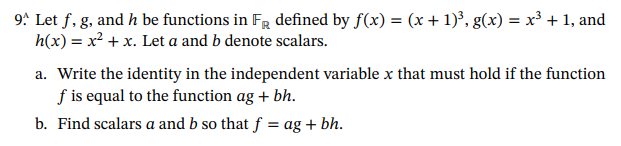# (Solved): 9. Let \( f, g \), and \( h \) be functions in \( \mathbb{F}_{\mathbb{R}} \) defined by \( f(x)=(x ...9. Let \( f, g \), and \( h \) be functions in \( \mathbb{F}_{\mathbb{R}} \) defined by \( f(x)=(x+1)^{3}, g(x)=x^{3}+1 \), and \( h(x)=x^{2}+x \). Let \( a \) and \( b \) denote scalars. a. Write the identity in the independent variable \( x \) that must hold if the function \( f \) is equal to the function \( a g+b h \). b. Find scalars \( a \) and \( b \) so that \( f=a g+b h \).

We have an Answer from Expert